Hydraulic Repairs & Sales
800-728-5168DON'T SEE WHAT YOU NEED ONLINE?

* Over 1,000,000 units not listed*

• CALL TOLL FREE
800-728-5168

Search
Quick Quote

Mobile App

Anytime, Anywhere

Request quotes from your phone through PFP's Quote App.

Get the App

# Hydraulic Accumulator Formulas

Formula For: Word Formula Letter Formula
Pressure or Volume
w/ Constant “T” (Temperature)
Original Pressure x Original Volume = Final Pressure x Final Volume P1 V1  =  P2 V2   Isothermic
Pressure or Temperature
w/ Constant “V” (Volume)
Original Pressure x Final Temperature = Final… Continue reading

## Hydraulic Capacity & Volume Equivalents

Cubic Inches Cubic Feet Cubic Yards
CU Inches 1. .0005787 .00002143
CU Feet 1,728. 1. .037037
CU Yards 46,656. 27. 1.
Liters 61.0234 .0353145 .001308
U.S. Gallons 231. .133681 .004951
Imp. Gallons 277.274 .160549 .0059429
Lbs Water 27.6798 .0160184 .0005929

# Thermal Formulas

Formula For: Word Formula Letter Formula
Reservoir Cooling Capacity
Heat (BTU/HR) =
2 x Temperature Difference Between Reservoir Walls And Air (ºF) x Area of Reservoir (Sq.Ft.)
BTU/Hr = 2.0 xT x A
Heat in Hydraulic Oil (approx.)
Due to System Inefficiency(SG=.89-.92)
Heat (BTU/HR) =

# Hydraulic Cylinder Formulas

Cylinder Formulas For:

Word Formula

Letter Formula

Cylinder Area
In Square Inches
Area =x Radius2 (Inches)
Area =x Diameter2 (Inches)
A =r2
A =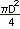or  A = .785D2
Cylinder Force
In Pounds, Push or Pull
Force = Pressure (psi) x Net Area (Square Inches)… Continue reading

# Hydraulic Pump Formulas

Pump Formulas For: Word Formula Letter Formula
Pump Outlet Flow
In Gallons/Minute
Flow =Q =Pump Input Power
In Horsepower Required
Horsepower Input =HPIN =orPump Efficiency
Overall in Percent
Overall Efficiency =X 100
Overall Efficiency = Volumetric Eff. x Mechanical Eff.
EffQVx… Continue reading

# Hydraulic Fluid Formulas

Hydraulic Fluid Formulas For: Word Formula Letter Formula
Velocity Through Piping
In Feet/Second Velocity
Velocity =V =Compressibility of Oil
In Additional Required Oil To Reach PressureVolume
VA =* Approximately 1/2 % per 100 psi
Compressibility of a Fluid Compressibility =C(ß)=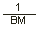## Hydraulic Formula Table

Formula For: Word Formula Letter Formula
Fluid Pressure
In Pounds/Square Inch
Pressure =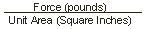P =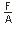or
psi =Fluid Flow Rate
In Gallons/Minute
Flow Rate =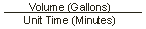Q =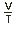Fluid Power
In Horsepower
Horsepower =HP =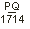MANUFACTURERS